# Creating plots in R using ggplot2 - part 10: boxplots

written in

This is the tenth tutorial in a series on using ggplot2 I am creating with Mauricio Vargas Sepúlveda. In this tutorial we will demonstrate some of the many options the ggplot2 package has for creating and customising boxplots. We will use R’s airquality dataset in the datasets package.

Mauricio and I have also published these graphing posts as a book on Leanpub. We tend to put any changes or updates to the code in the book before these blog posts, so please check it out if you have any issues with the code examples in this post; otherwise feel free to contact us with any questions!

The first thing to do is load in the data, as below. We’ll convert Month into a labelled factor in order to use it as our grouping variable.

rm(list = ls())
library(datasets)
library(ggplot2)

data(airquality)
airquality$Month <- factor(airquality$Month,
labels = c("May", "Jun", "Jul", "Aug", "Sep"))


In this tutorial, we will work towards creating the boxplot below. We will take you from a basic boxplot and explain all the customisations we add to the code step-by-step.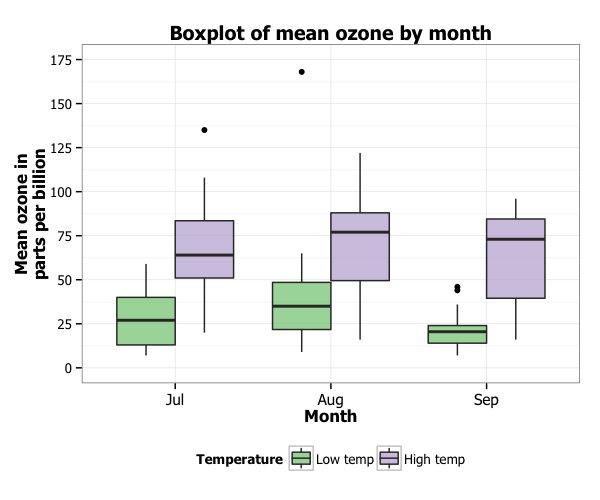### Basic boxplot

In order to initialise a plot we tell ggplot that airquality is our data, and specify that our x-axis plots the Month variable and our y-axis plots the Ozone variable. We then instruct ggplot to render this as a boxplot by adding the geom_boxplot() option.

p10 <- ggplot(airquality, aes(x = Month, y = Ozone)) +
geom_boxplot()
p10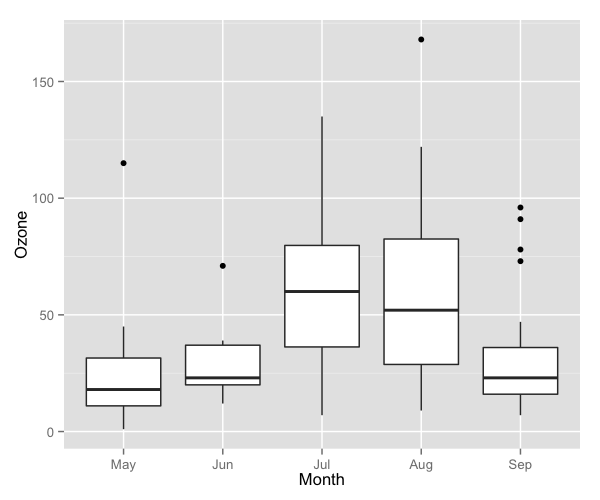### Customising axis labels

In order to change the axis labels, we have a couple of options. In this case, we have used the scale_x_discrete and scale_y_continuous options, as these have further customisation options for the axes we will use below. In each, we add the desired name to the name argument as a string.

p10 <- p10 + scale_x_discrete(name = "Month") +
scale_y_continuous(name = "Mean ozone in parts per billion")
p10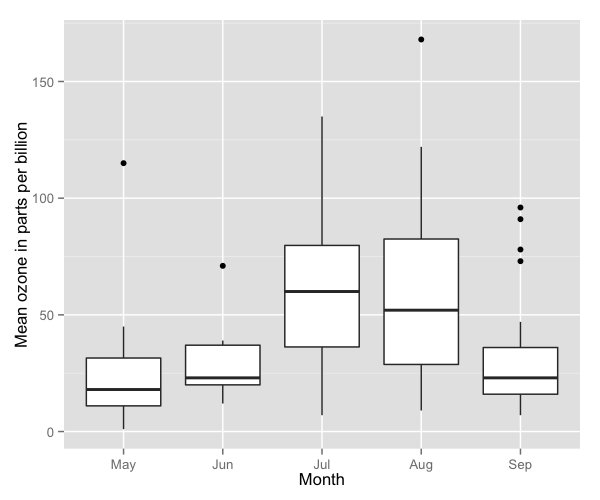ggplot also allows for the use of multiline names (in both axes and titles). Here, we’ve changed the y-axis label so that it goes over two lines using the \n character to break the line.

p10 <- p10 + scale_y_continuous(name = "Mean ozone in\nparts per billion")
p10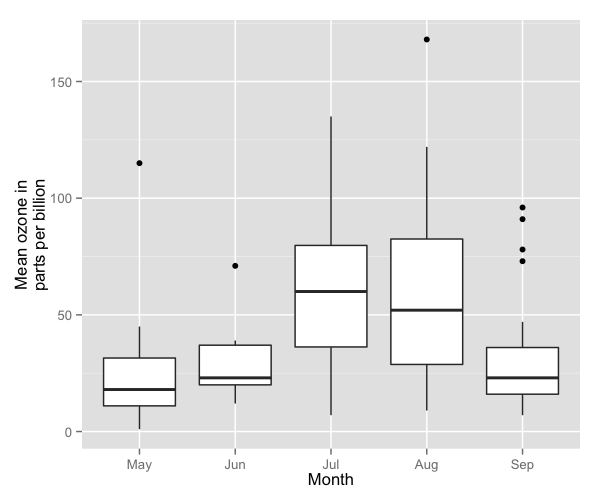### Changing axis ticks

The next thing we will change is the axis ticks. Let’s make the y-axis ticks appear at every 25 units rather than 50 using the breaks = seq(0, 175, 25) argument in scale_y_continuous. (The seq function is a base R function that indicates the start and endpoints and the units to increment by respectively. See help(seq) for more information.) We ensure that the y-axis begins and ends where we want by also adding the argument limits = c(0, 175) to scale_y_continuous.

p10 <- p10 + scale_y_continuous(name = "Mean ozone in\nparts per billion",
breaks = seq(0, 175, 25),
limits=c(0, 175))
p10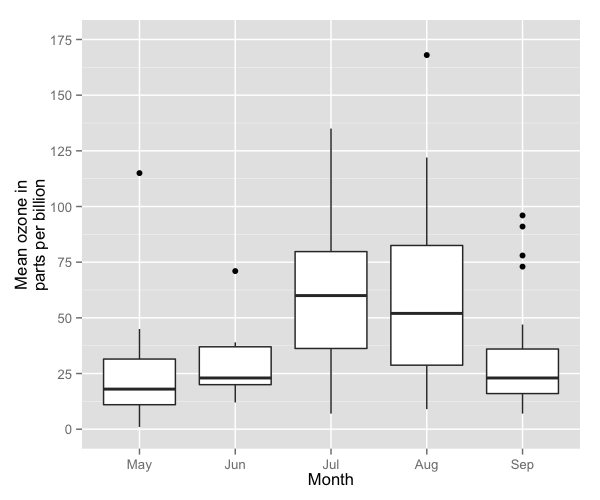To add a title, we include the option ggtitle and include the name of the graph as a string argument.

p10 <- p10 + ggtitle("Boxplot of mean ozone by month")
p10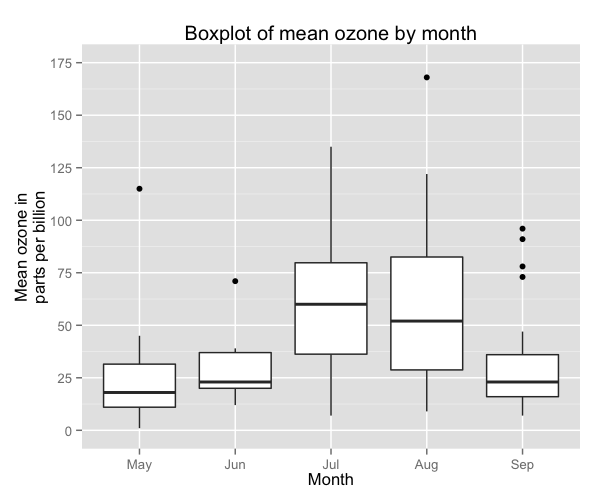### Changing the colour of the boxes

To change the line and fill colours of the box plot, we add a valid colour to the colour and fill arguments in geom_boxplot() (note that we assigned these colours to variables outside of the plot to make it easier to change them). A list of valid colours is here.

fill <- "gold1"
line <- "goldenrod2"

p10 <- ggplot(airquality, aes(x = Month, y = Ozone)) +
geom_boxplot(fill = fill, colour = line) +
scale_y_continuous(name = "Mean ozone in\nparts per billion",
breaks = seq(0, 175, 25),
limits=c(0, 175)) +
scale_x_discrete(name = "Month") +
ggtitle("Boxplot of mean ozone by month")
p10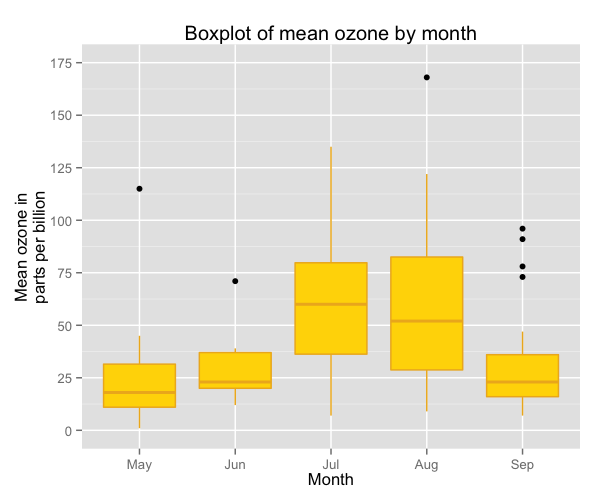If you want to go beyond the options in the list above, you can also specify exact HEX colours by including them as a string preceded by a hash, e.g., “#FFFFFF”. Below, we have called two shades of blue for the fill and lines using their HEX codes.

fill <- "#4271AE"
line <- "#1F3552"

p10 <- ggplot(airquality, aes(x = Month, y = Ozone)) +
geom_boxplot(fill = fill, colour = line) +
scale_y_continuous(name = "Mean ozone in\nparts per billion",
breaks = seq(0, 175, 25),
limits=c(0, 175)) +
scale_x_discrete(name = "Month") +
ggtitle("Boxplot of mean ozone by month")
p10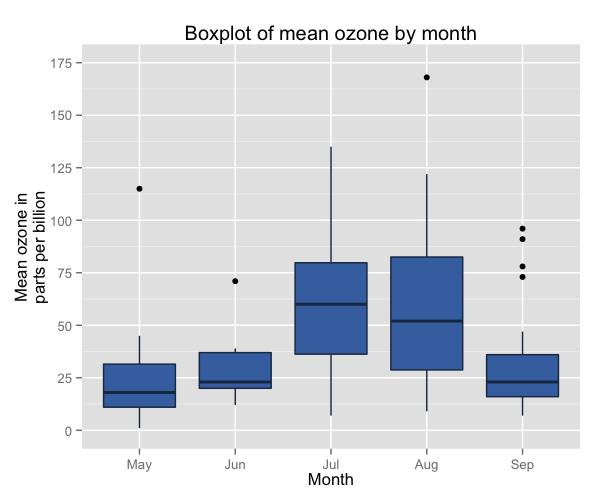You can also specify the degree of transparency in the box fill area using the argument alpha in geom_boxplot. This ranges from 0 to 1.

p10 <- ggplot(airquality, aes(x = Month, y = Ozone)) +
geom_boxplot(fill = fill, colour = line,
alpha = 0.7) +
scale_y_continuous(name = "Mean ozone in\nparts per billion",
breaks = seq(0, 175, 25),
limits=c(0, 175)) +
scale_x_discrete(name = "Month") +
ggtitle("Boxplot of mean ozone by month")
p10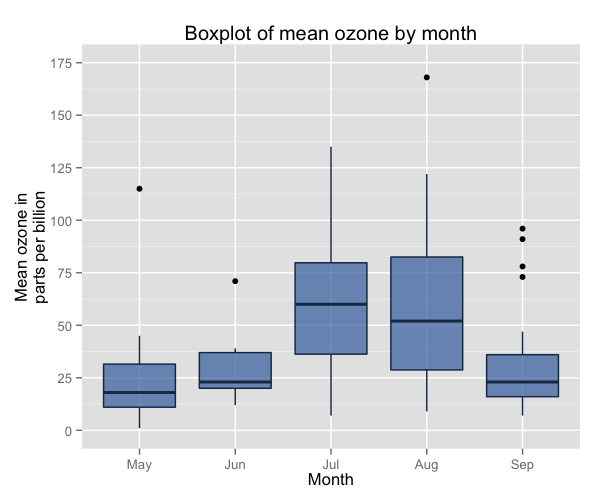Finally, you can change the appearance of the outliers as well, using the arguments outlier.colour and outlier.shape in geom_boxplot to change the colour and shape respectively. An explanation of the allowed arguments for shape are described in this article, although be aware that because there is no “fill” argument for outlier, you cannot create circles with separate outline and fill colours. Here we will make the outliers small solid circles (using outlier.shape = 20) and make them the same colour as the box lines (using outlier.colour = "#1F3552").

p10 <- ggplot(airquality, aes(x = Month, y = Ozone)) +
geom_boxplot(fill = fill, colour = line, alpha = 0.7,
outlier.colour = "#1F3552", outlier.shape = 20) +
scale_y_continuous(name = "Mean ozone in\nparts per billion",
breaks = seq(0, 175, 25),
limits=c(0, 175)) +
scale_x_discrete(name = "Month") +
ggtitle("Boxplot of mean ozone by month")
p10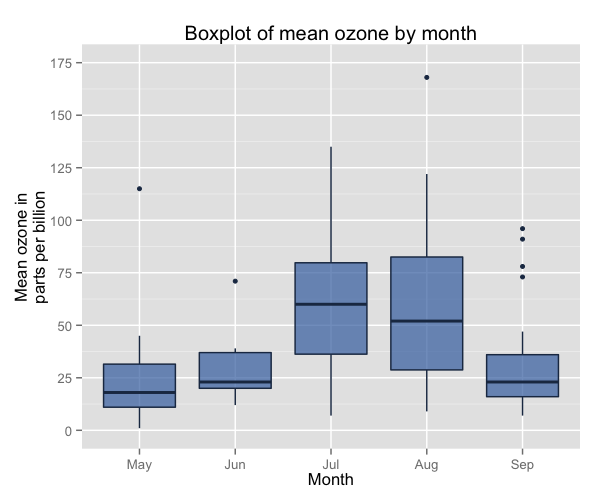### Using the white theme

As explained in the previous posts, we can also change the overall look of the plot using themes. We’ll start using a simple theme customisation by adding theme_bw(). As you can see, we can further tweak the graph using the theme option, which we’ve used so far to change the legend.

p10 <- p10 + theme_bw()
p10### Creating an XKCD style chart

Of course, you may want to create your own themes as well. ggplot2 allows for a very high degree of customisation, including allowing you to use imported fonts. Below is an example of a theme Mauricio was able to create which mimics the visual style of XKCD. In order to create this chart, you first need to import the XKCD font, install it on your machine and load it into R using the extrafont package. These instructions are taken from here:

library(extrafont)

dest="xkcd.ttf", mode="wb")
system("mkdir ~/.fonts")
system("cp xkcd.ttf  ~/.fonts")
font_import(paths = "~/.fonts", pattern="[X/x]kcd")
fonts()


You can then create your graph:

p10 <- ggplot(airquality, aes(x = Month, y = Ozone)) +
geom_boxplot(colour = "black", fill = "#56B4E9") +
scale_y_continuous(name = "Mean ozone in\nparts per billion",
breaks = seq(0, 175, 25),
limits=c(0, 175)) +
scale_x_discrete(name = "Month") +
ggtitle("Boxplot of mean ozone by month") +
theme(axis.line.x = element_line(size = 0.5, colour = "black"),
axis.line.y = element_line(size = 0.5, colour = "black"),
axis.line = element_line(size=1, colour = "black"),
panel.grid.major = element_blank(),
panel.grid.minor = element_blank(),
panel.border = element_blank(),
panel.background = element_blank(),
plot.title=element_text(size = 20, family="xkcd-Regular"),
text=element_text(size = 16, family="xkcd-Regular"),
axis.text.x=element_text(colour="black", size = 12),
axis.text.y=element_text(colour="black", size = 12))
p10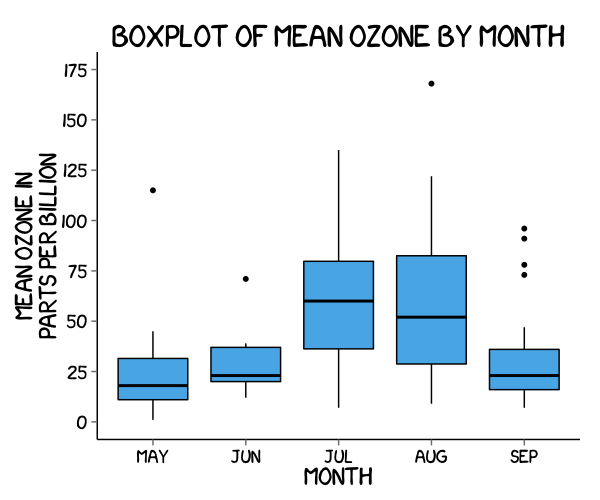### Using ‘The Economist’ theme

There are a wider range of pre-built themes available as part of the ggthemes package (more information on these here). Below we’ve applied theme_economist(), which approximates graphs in the Economist magazine.

library(ggthemes)
library(grid)

fill <- "#4271AE"
line <- "#1F3552"

p10 <- ggplot(airquality, aes(x = Month, y = Ozone)) +
geom_boxplot(fill = fill, colour = line) +
scale_y_continuous(name = "Mean ozone in\nparts per billion",
breaks = seq(0, 175, 25),
limits=c(0, 175)) +
scale_x_discrete(name = "Month") +
ggtitle("Boxplot of mean ozone by month") +
theme_economist() +
theme(axis.line.x = element_line(size = 0.5, colour = "black"),
axis.line.y = element_line(size = 0.5, colour = "black"),
legend.position = "bottom", legend.direction = "horizontal",
legend.box = "horizontal",
legend.key.size = unit(1, "cm"),
plot.title = element_text(family="Tahoma"),
text = element_text(family = "Tahoma"),
axis.title = element_text(size = 12),
legend.text = element_text(size = 9),
legend.title=element_text(face = "bold", size = 9))
p10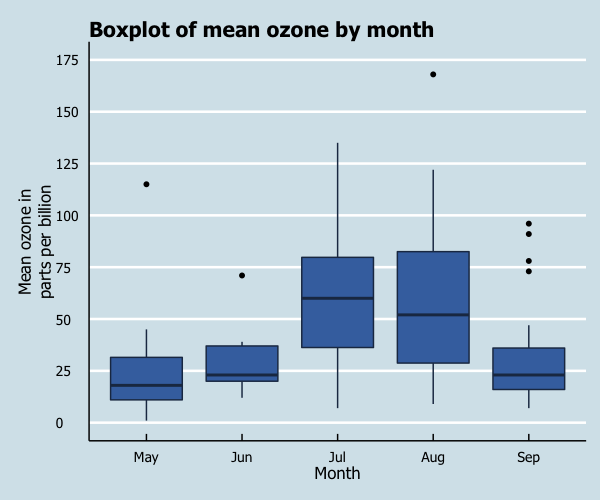As before, you can modify your plots a lot as ggplot2 allows many customisations. Here is a custom plot where we have modified the axes, background and font.

library(grid)

fill <- "#4271AE"
lines <- "#1F3552"

p10 <- ggplot(airquality, aes(x = Month, y = Ozone)) +
geom_boxplot(colour = lines, fill = fill,
size = 1) +
scale_y_continuous(name = "Mean ozone in\nparts per billion",
breaks = seq(0, 175, 25),
limits=c(0, 175)) +
scale_x_discrete(name = "Month") +
ggtitle("Boxplot of mean ozone by month") +
theme_bw() +
theme(panel.grid.major = element_line(colour = "#d3d3d3"),
panel.grid.minor = element_blank(),
panel.border = element_blank(),
panel.background = element_blank(),
plot.title = element_text(size = 14, family = "Tahoma", face = "bold"),
text=element_text(family = "Tahoma"),
axis.title = element_text(face="bold"),
axis.text.x = element_text(colour="black", size = 11),
axis.text.y = element_text(colour="black", size = 9),
axis.line = element_line(size=0.5, colour = "black"))
p10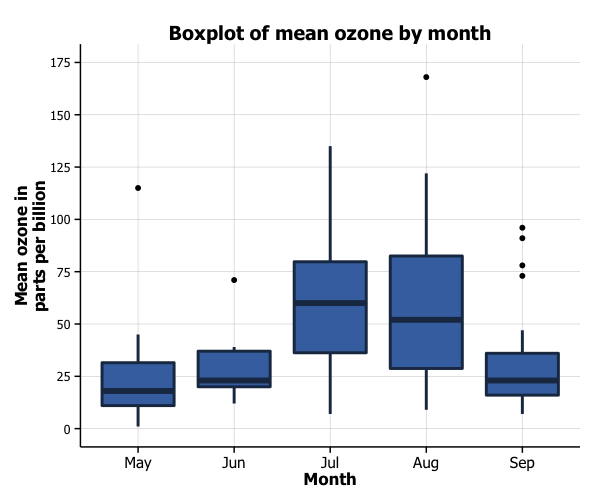### Boxplot extras

An extra feature you can add to boxplots is to overlay all of the points for that group on each boxplot in order to get an idea of the sample size of the group. This can be achieved using by adding the geom_jitter option.

p10 <- p10 + geom_jitter()
p10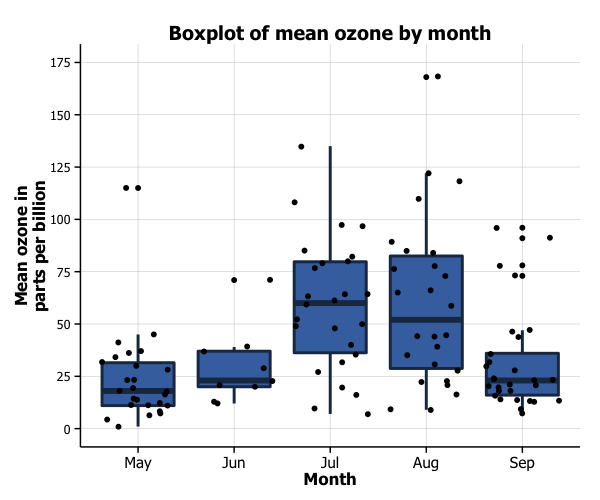We can see that June has a pretty small sample, indicating that information based on this group may not be very reliable.

Another thing you can do with your boxplot is add a notch to the box where the median sits to give a clearer visual indication of how the data are distributed within the IQR. You achieve this by adding the argument notch = TRUE to the geom_boxplot option. You can see on our graph that the box for June looks a bit weird due to the very small gap between the 25th percentile and the median.

p10 <- ggplot(airquality, aes(x = Month, y = Ozone)) +
geom_boxplot(colour = lines, fill = fill,
size = 1, notch = TRUE) +
scale_y_continuous(name = "Mean ozone in\nparts per billion",
breaks = seq(0, 175, 25),
limits=c(0, 175)) +
scale_x_discrete(name = "Month") +
ggtitle("Boxplot of mean ozone by month") +
theme_bw() +
theme(panel.grid.major = element_line(colour = "#d3d3d3"),
panel.grid.minor = element_blank(),
panel.border = element_blank(), panel.background = element_blank(),
plot.title = element_text(size = 14, family = "Tahoma", face = "bold"),
text=element_text(family="Tahoma"),
axis.title = element_text(face="bold"),
axis.text.x=element_text(colour="black", size = 11),
axis.text.y=element_text(colour="black", size = 9),
axis.line = element_line(size=0.5, colour = "black"))
p10### Grouping by another variable

You can also easily group box plots by the levels of another variable. There are two options, in separate (panel) plots, or in the same plot.

We first need to do a little data wrangling. In order to make the graphs a bit clearer, we’ve kept only months “July”, “Aug” and “Sep” in a new dataset airquality_trimmed. We’ve also mean-split Temp so that this is also categorical, and made it into a new labelled factor variable called Temp.f.

In order to produce a panel plot by temperature, we add the facet_grid(. ~ Temp.f) option to the plot.

airquality_trimmed <- airquality[which(airquality$Month == "Jul" | airquality$Month == "Aug" |
airquality$Month == "Sep"), ] airquality_trimmed$Temp.f <- factor(ifelse(airquality_trimmed$Temp > mean(airquality_trimmed$Temp), 1, 0),
labels = c("Low temp", "High temp"))

p10 <- ggplot(airquality_trimmed, aes(x = Month, y = Ozone)) +
geom_boxplot(fill = fill, colour = line,
alpha = 0.7) +
scale_y_continuous(name = "Mean ozone in\nparts per billion",
breaks = seq(0, 175, 50),
limits=c(0, 175)) +
scale_x_discrete(name = "Month") +
ggtitle("Boxplot of mean ozone by month") +
theme_bw() +
theme(plot.title = element_text(size = 14, family = "Tahoma", face = "bold"),
text = element_text(size = 12, family = "Tahoma"),
axis.title = element_text(face="bold"),
axis.text.x=element_text(size = 11)) +
facet_grid(. ~ Temp.f)
p10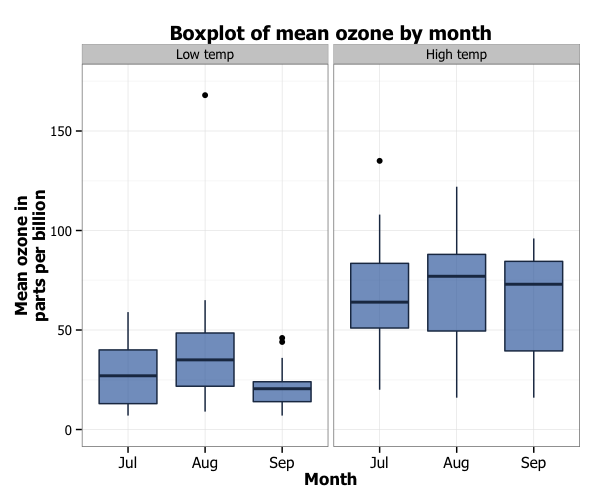In order to plot the two temperature levels in the same plot, we need to add a couple of things. Firstly, in the ggplot function, we add a fill = Temp.f argument to aes. Secondly, we customise the colours of the boxes by adding the scale_fill_brewer to the plot from the RColorBrewer package. This blog post describes the available packages.

library(RColorBrewer)

p10 <- ggplot(airquality_trimmed, aes(x = Month, y = Ozone, fill = Temp.f)) +
geom_boxplot(alpha=0.7) +
scale_y_continuous(name = "Mean ozone in\nparts per billion",
breaks = seq(0, 175, 25),
limits=c(0, 175)) +
scale_x_discrete(name = "Month") +
ggtitle("Boxplot of mean ozone by month") +
theme_bw() +
theme(plot.title = element_text(size = 14, family = "Tahoma", face = "bold"),
text = element_text(size = 12, family = "Tahoma"),
axis.title = element_text(face="bold"),
axis.text.x=element_text(size = 11)) +
scale_fill_brewer(palette = "Accent")
p10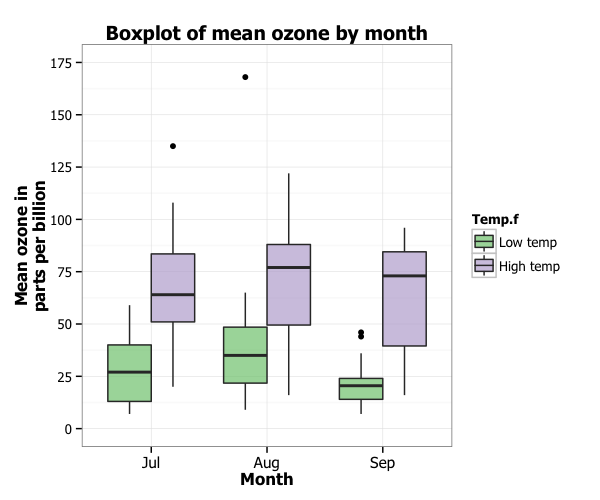### Formatting the legend

Finally, we can format the legend. Firstly, we can change the position by adding the legend.position = "bottom" argument to the theme option, which moves the legend under the plot. Secondly, we can fix the title by adding the labs(fill = "Temperature") option to the plot.

p10 <- ggplot(airquality_trimmed, aes(x = Month, y = Ozone, fill = Temp.f)) +
geom_boxplot(alpha=0.7) +
scale_y_continuous(name = "Mean ozone in\nparts per billion",
breaks = seq(0, 175, 25),
limits=c(0, 175)) +
scale_x_discrete(name = "Month") +
ggtitle("Boxplot of mean ozone by month") +
theme_bw() +
theme(plot.title = element_text(size = 14, family = "Tahoma", face = "bold"),
text = element_text(size = 12, family = "Tahoma"),
axis.title = element_text(face="bold"),
axis.text.x=element_text(size = 11),
legend.position = "bottom") +
scale_fill_brewer(palette = "Accent") +
labs(fill = "Temperature")
p10